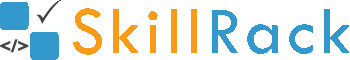Left Shift Operator1 year ago

Left Shift operator is denoted by <<

<< shifts the bits to the left by the denoted positions.

N << 1 shifts the bits in N to the left by 1 position.

Consider 9 whose binary representation is 1001.

Now when 1001 is shifted left using << by 1, we get 10010 (a zero is added in the end).

Decimal value of 10010 is 18.

So the below program prints 18 as the output.

#include <stdio.h>

int main(){
//Binary representation of 9 is 1001
int N = 9;

/*
Now 1001 is shifted left using << by 1.
So it becomes 10010.  Decimal value of 10010 is 18.
*/
printf("%d", N << 1);
}

Another Example:

Consider N=6. Binary representation of 6 is 110. Now 110 is shifted left using << by 2 giving the value as 11000.  Decimal value of 11000 is 24.

So the output of the program is 24.

#include <stdio.h>

int main(){
//Binary representation of 6 is 110
int N = 6;

/*
Now 110 is shifted left using << by 2.
So it becomes 11000.  Decimal value of 11000 is 24.
*/
printf("%d", N << 2);
}